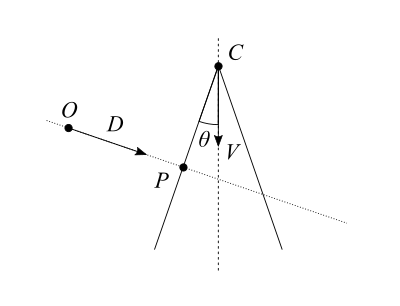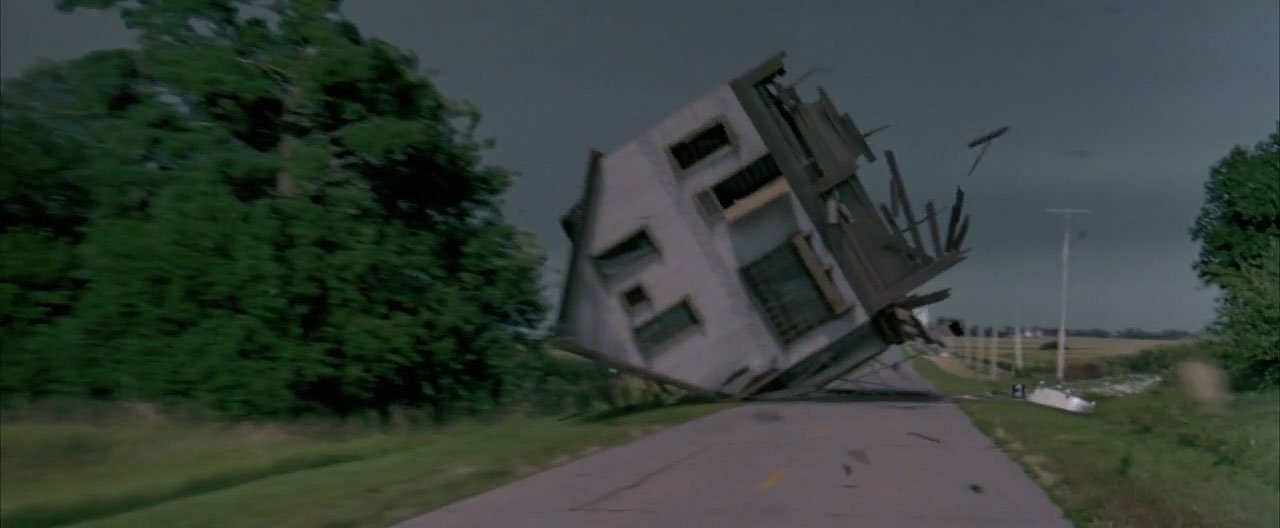# Intersection of a ray and a cone

Some time ago I needed to solve analytically the intersection of a ray and a cone. I was surprised to see that there are not that many resources available; there are some, but not nearly as many as on the intersection of a ray and a sphere for example. Add to it that they all use their own notation and that I lack math exercise, after a bit of browsing I decided I needed to write a proof by myself to get a good grasp of the result.

So here goes, the solution to the intersection of a ray and a cone, in vector notation.

1. We define a ray with its origin $O$ and its direction as a unit vector $\hat{D}$.
Any point $X$ on the ray at a signed distance $t$ from the origin of the ray verifies: $\vec{X} = \vec{O} + t\vec{D}$.
When $t$ is positive $X$ is in the direction of the ray, and when $t$ is negative $X$ is in the opposite direction.
2. We define a cone with its tip $C$, its axis as a unit vector $\hat{V}$ in the direction of increasing radius, and $\theta$ the half angle between the axis and the surface.
Any point $X$ on the cone verifies: $(\vec{X} – \vec{C}) \cdot \vec{V} = \lVert \vec{X} – \vec{C} \rVert \cos\theta$
3. Finally we define $P$ the intersection or the ray and the cone, and which we are interested in finding.$P$ verifies both equations, so we can write:

$$\left\{ \begin{array}{l} \vec{P}=\vec{O} + t\vec{D} \\ \frac{ \vec{P} – \vec{C} }{\lVert \vec{P} – \vec{C} \rVert} \vec{V} = \cos\theta \end{array} \right.$$

We can multiply the second equation by itself to work with it, then reorder things a bit.

$$\left\{ \begin{array}{l} \vec{P}=\vec{O} + t\vec{D} \\ \frac{ ((\vec{P} – \vec{C}) \cdot \vec{V})^2 }{ (\vec{P} – \vec{C}) \cdot (\vec{P} – \vec{C}) } = \cos^2\theta \end{array} \right.$$

$$\left\{ \begin{array}{l} \vec{P}=\vec{O} + t\vec{D} \\ ((\vec{P} – \vec{C}) \cdot \vec{V})^2 – (\vec{P} – \vec{C}) \cdot (\vec{P} – \vec{C}) \cos^2\theta = 0 \end{array} \right.$$

Remember the mouthful earlier about $\hat{V}$ being in the direction of increasing radius? By elevating $\cos\theta$ to square, we’re making negative values of $\cos$ positive: values of $\theta$ beyond 90° become indistinguishable from values below 90°. This has the side effect of turning it into the equation of not one, but two cones sharing the same axis, tip and angle, but in opposite directions. We’ll fix that later.

We replace $\vec{P}$ with $\vec{O} + t\vec{D}$ and work the equation until we get a good old quadratic function that we can solve.

$$\require{cancel} ((\vec{O} + t\vec{D} – \vec{C})\cdot\vec{V})^2 – (\vec{O} + t\vec{D} – \vec{C}) \cdot (\vec{O} + t\vec{D} – \vec{C}) \cos^2\theta = 0$$

$$((t\vec{D} + \vec{CO})\cdot\vec{V})^2 – (t\vec{D} + \vec{CO}) \cdot (t\vec{D} + \vec{CO}) \cos^2\theta = 0$$

$$(t\vec{D}\cdot\vec{V} + \vec{CO}\cdot\vec{V})^2 – (t^2\cancel{\vec{D}\cdot\vec{D}} + 2t\vec{D}\cdot\vec{CO} + \vec{CO}\cdot\vec{CO}) \cos^2\theta = 0$$

$$(t^2(\vec{D}\cdot\vec{V})^2 + 2t(\vec{D}\cdot\vec{V})(\vec{CO}\cdot\vec{V}) + (\vec{CO}\cdot\vec{V})^2) – (t^2 + 2t\vec{D}\cdot\vec{CO} + \vec{CO}\cdot\vec{CO}) \cos^2\theta = 0$$

$$t^2(\vec{D}\cdot\vec{V})^2 + 2t(\vec{D}\cdot\vec{V})(\vec{CO}\cdot\vec{V}) + (\vec{CO}\cdot\vec{V})^2 – t^2\cos^2\theta – 2t\vec{D}\cdot\vec{CO}\cos^2\theta – \vec{CO}\cdot\vec{CO}\cos^2\theta = 0$$

Reorder a bit:

$$t^2((\vec{D}\cdot\vec{V})^2 – \cos^2\theta) + 2t((\vec{D}\cdot\vec{V})(\vec{CO}\cdot\vec{V}) – \vec{D}\cdot\vec{CO}\cos^2\theta) + (\vec{CO}\cdot\vec{V})^2 – \vec{CO}\cdot\vec{CO}\cos^2\theta = 0$$

There we go, we have our $at^2 + bt + c = 0$ equation, with:

$$\left\{ \begin{array}{l} a = (\vec{D}\cdot\vec{V})^2 – \cos^2\theta \\ b = 2\Big((\vec{D}\cdot\vec{V})(\vec{CO}\cdot\vec{V}) – \vec{D}\cdot\vec{CO}\cos^2\theta\Big) \\ c = (\vec{CO}\cdot\vec{V})^2 – \vec{CO}\cdot\vec{CO}\cos^2\theta \end{array} \right.$$

From there, you know the drill: calculate the determinant $\Delta = b^2 – 4ac$ then depending on its value:

• If $\Delta < 0$, the ray is not intersecting the cone.
• If $\Delta = 0$, the ray is intersecting the cone once at $t = \frac{-b}{2a}$.
• If $\Delta > 0$, the ray is intersecting the cone twice, at $t_1 = \frac{-b – \sqrt{\Delta}}{2a}$ and $t_2 = \frac{-b + \sqrt{\Delta}}{2a}$.

But wait! We don’t have one cone but two, so we have to reject solutions that intersect with the shadow cone. $P$ must still verify $\frac{ \vec{P} – \vec{C} }{\lVert \vec{P} – \vec{C} \rVert} \vec{V} = \cos\theta$, or simply, if $\theta < 90°$: $(\vec{P} – \vec{C})\cdot\vec{V} > 0$.

Note that there is also the corner case of the ray tangent to the cone and having an infinity of solutions to consider. I’ve completely swept it under the rug since it doesn’t matter in the context I was, but if it does to you, you’ve been warned about it. Also remember to check the sign of $t$ to know whether $P$ is in the direction of the ray. You may need to determine which of $t_1$ or $t_2$ you want to use, which depends on your use case. For example is your ray origin inside or outside of the cone?

Now for a little sanity test, let’s consider the corner case $C=O$, where the ray origin is the tip of the cone (thanks Rubix for the suggestion!). We have $b=0$ and $c=0$ thus $\Delta=0$ and $t=\frac{-b}{2a}=0$ which is the expected result.

I also tried the cases $\theta=0$ and $\theta=\pi/2$, but expanding $\Delta$ proved too tedious to proceed to the end. So this is left as an exercise, as they say. :)

Finally, to demonstrate that the result is indeed correct, here is a glorious ray traced cone scene on ShaderToy:

I hope this can prove useful to others too.

Oh, and Happy New Year by the way!

# Sophie Wilson – The future of microprocessors

In this 2014 talk, one of the designers of the original ARM processor, Sophie Wilson, gives an overview of the history of processors and what to expect in future.

The presentation covers in layman’s terms topics like Moore’s law (obviously), pipelining, parallelism, power consumption, heat dissipation, processor specialization and cost of production among other things. As explained, all those aspects are facing difficult challenges that are likely to shape the future of microprocessors, which in turns impacts both hardware and software engineers.

Here is the same presentation in 2016:

# Francesca Da Rimini

Following yesterday’s post about a music video featuring modern dance and computer visual effects, here is a video featuring classical dance and a robot controlled camera.

Francesca Da Rimini was a historical figure portrayed in the Divine Comedy and numerous works of art, including a symphonic poem by Tchaikovsky. In 2014 the director Tarik Abdel-Gawad and his team recorded a performance by two dancers of the San Francisco Ballet, Maria Kochetkova and Joan Boada, using a robot controlled camera. Tarik was also the technical and creative director of the demonstration video featuring the same Bot&Dolly robots (a company acquired by Google in 2013) and which turned viral, “Box”.

In the accompanying back stage video, he explains how seeing dancers rehearse over and over gave him the idea of experimenting with a pre-programmed robot, in order to make the camera part of the choregraphy, and allow the viewer to have a closer, more intimate, view of the performance.

# The Chemical Brothers: “Wide Open”

Earlier this year at Tokyo Demo Fest 2016, we were honored with the presence of Solid Angle‘s founder, Marcos Fajardo, who did a long presentation of the Arnold renderer. Various examples of productions that involved Arnold were included, like captures from Iron Man or Elysium, but between those blockbusters one production in particular held my attention.It was a long shot of a dancer turning limb after limb into a lattice body while performing. That film is in fact the music video of the song “Wide Open”, by the Chemical Brothers, and directed by Dom&Nic. It is a brilliant piece of technical and artistic work, that I can only recommend to watch.

Some of the details of the creation are shared in an article by the excellent fxguide, as well as in this interview for Solid Angle, like for example how they dealt with the challenges posed by a single long shot under varying natural light. Finally, there is this behind the scenes video from the studio, The Mill:

# Making of the “spinning house” sequence in Twister (1996)Earlier this year filmmaker Stu Maschwitz posted on Twitter a series of messages recounting the work he did, as a then junior visual effects artist, on the scene of the house crashing on the road in the 1996 summer blockbuster, Twister. He later copied them to his website: go read it there.

The story comes with a fair amount of detail, hacks and tricks to make the best of the technical limitations of the time, and gives an idea of the amount of work such a scene in a prominent Hollywood film entails.

# Metal materials study

Texture artist Jarrod Hasenjager posted a page of various metal materials study: aluminum, brass, bronze, chrome, copper, gold, iron, lead and steel, and rusted steel and iron. According to the description, the renders are done in Houdini, and the look is driven by artistic taste and personal experience rather than from physical values.